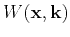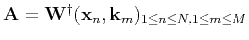Seismic wave extrapolation using lowrank symbol approximationNext: EXAMPLES Up: Fomel, Ying, & Song: Previous: WAVE EXTRAPOLATION

# LOWRANK APPROXIMATION

The key idea of the lowrank decomposition is decomposing the wave extrapolation matrix(11)

for a fixedinto a separated representation(12)

Representation (12) speeds up the computation ofsince(13)

The evaluation of the last formula is effectively equivalent to applyinginverse Fast Fourier Transforms. Physically, a separable lowrank approximation amounts to selecting a set ofrepresentative spatial locations andrepresentative wavenumbers.

In order to discuss the construction of approximation (12), let us view it as a matrix decomposition problem(14)

whereis thematrix with entries,is the submatrix ofthat consists of the columns associated with,is the submatrix that consists of the rows associated with, and. In practice, we find that the matrixhas a low rank separated representation provided thatis sufficiently small, which, in the case of smooth models, can be partially explained by the separation of terms in the Taylor series 5. Letbe a prescribed accuracy of this separated representation, andbe the numerical rank of. The construction of the separated representation in equation (14) follows the method of Engquist and Ying (2007, 2009) and is detailed in the appendix. The main observation is that the columns ofand the rows ofshould span the column space and row space of, respectively, as well as possible. The algorithm for computing (14) takes the following steps:
1. Pick a uniformly random setofcolumns ofwhereis chosen to be 3 or 4 in practice. Perform the pivoted QR factorization to(Golub and Van Loan, 1996). The firstpivoted columns correspond torows of the matrix. Defineto be the submatrix ofthat consists of these rows and setwithto be the correspondingvalues of these rows.
2. Pick a uniformly random setofrows ofand perform the pivoted QR factorization to. Defineto be the submatrix ofthat consists of these columns and setwithto be the correspondingvalues of these columns.
3. Set the middle matrixwherestands for the pseudoinverse.
4. Combining the result of the previous three steps gives the required separated representation.
The algorithm does not require, at any step, access to the full matrix, only to its selected rows and columns. Once the decomposition is complete, it can be used at every time step during the wave extrapolation process. In multiple-core implementations, the matrix operations in equation (12) are easy to parallelize. The algorithm details are outlined in the appendix.

The cost of the algorithm isoperations per time step, whererefers to the cost of the Fourier transform. In comparison, the cost of finite-difference wave extrapolation is, whereis the size of the finite-difference stencil. Song et al. (2011) present an application of the proposed lowrank approximation algorithm for devising accurate finite-different schemes. There is a natural trade-off in the selection of: larger values lead to a more accurate wave representation but require a longer computational time. In the examples of the next section, we select these parameters based on an estimate of the approximation accuracy and generally aiming for the relative accuracy of. The resultingis typically smaller than the number of Fourier transforms required for pseudo-spectral algorithms such as pseudo-spectral implementations of the rapid expansion method (Pestana and Stoffa, 2011).Seismic wave extrapolation using lowrank symbol approximationNext: EXAMPLES Up: Fomel, Ying, & Song: Previous: WAVE EXTRAPOLATION

2013-08-31